# NCERT Solutions For Class 10 Maths Chapter 6 Triangles Ex 6.2

Get Free NCERT Solutions for Class 10 Maths Chapter 6 Ex 6.2 PDF. Triangles Class 10 Maths NCERT Solutions are extremely helpful while doing your homework. Exercise 6.2 Class 10 Maths NCERT Solutions were prepared by Experienced ncert-books.inTeachers. Detailed answers of all the questions in Chapter 6 Maths Class 10 Triangles Exercise 6.2 provided in NCERT TextBook.

You can also download the free PDF of Ex 6.2 Class 10 Triangles NCERT Solutions or save the solution images and take the print out to keep it handy for your exam preparation.

## NCERT Solutions For Class 10 Maths Chapter 6 Triangles Ex 6.2

NCERT Solutions for Class 10 Maths Chapter 6 Triangles Ex Ex 6.2 are part of NCERT Solutions for Class 10 Maths. Here we have given NCERT Solutions for Class 10 Maths Chapter 6 Triangles Exercise 6.2

Question 1.
In the given figure (i) and (ii), DE || BC. Find EC in (i) and AD in (ii).Solution: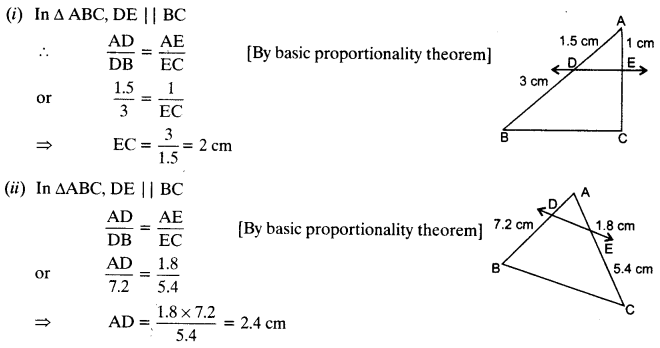Question 2.
E and F are points on the sides PQ and PR respectively of a ∆PQR. For each of the following cases, state whether EF || QR:
(i) PE = 3.9 cm, EQ = 3 cm, PF = 3.6 cm and FR = 2.4 cm
(ii) PE = 4 cm, QE = 4.5 cm, PF = 8 cm and RF = 9 cm
(iii) PQ = 1.28 cm, PR = 2.56 cm, PE = 0.18 cm and PF = 0.36 cm
Solution: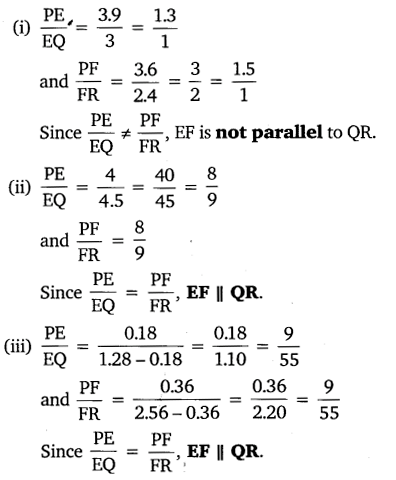Question 3.
In the given figure, if LM || CB and LN || CD.
Prove that$\frac { AM }{ AB } =\frac { AN }{ A{ D }^{ \bullet } }$Solution:Question 4.
In the given figure, DE || AC and DF || AE.
Prove that$\frac { BF }{ FE } =\frac { BE }{ E{ C }^{ \bullet } }$Solution: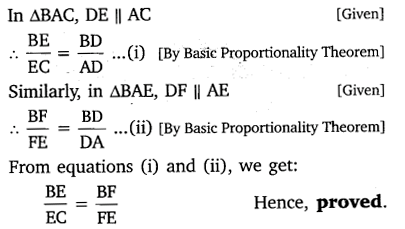Question 5.
In the given figure, DE || OQ and DF || OR. Show that EF || QR.Solution: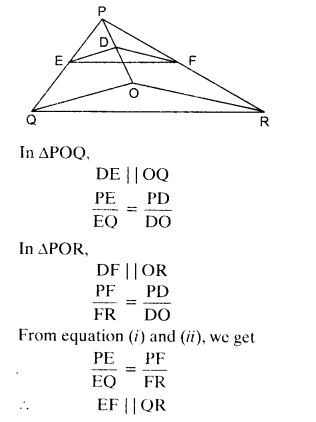Question 6.
In the given figure, A, B and C are points on OP, OQ and OR respectively such that AB || PQ and AC || PR. Show that BC || QR.Solution: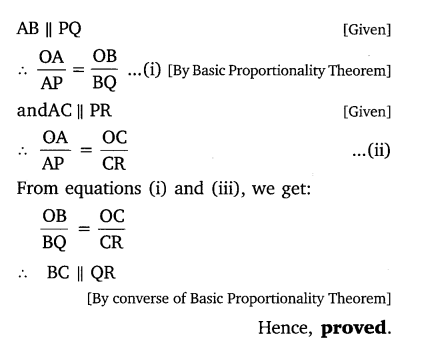Question 7.
Using B.P.T., prove that a line drawn through the mid-point of one side of a triangle parallel to another side bisects the third side. (Recall that your have proved it in class IX)
Solution:Question 8.
Using converse of B.P.T., prove that the line joining the mid-points of any two sides of a triangle is parallel to the third side.  (Recall that your have done it in class IX)
Solution: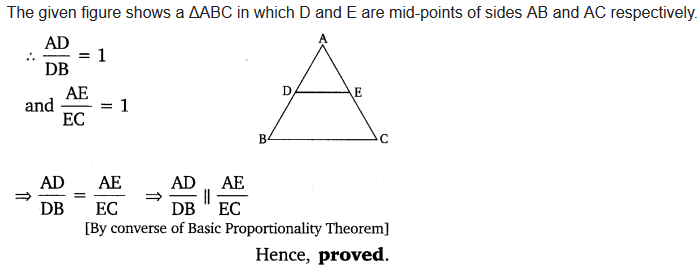Question 9.
ABCD is a trapezium in which AB || DC and its diagonals intersect each other at the point O. Show that$\frac { AO }{ BO } =\frac { CO }{ D{ O }^{ \bullet } }$
Solution:Question 10.
The diagonals of a quadrilateral ABCD intersect each other at the point O such that$\frac { AO }{ BO } =\frac { CO }{ D{ O }^{ \bullet } }$Show that ABCD is a trapezium.
Solution:error: Content is protected !!
+